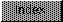```

NAME
SPSub -- Subtract two floating point numbers.

SYNOPSIS
fnum3 = SPSub(fnum1, fnum2)
D0            D1     D0

float SPSub(float fnum1, float fnum2);

FUNCTION
Accepts two floating point numbers and returns the arithmetic
subtraction of said numbers.

INPUTS
fnum1   - floating point number.
fnum2   - floating point number.

RESULT
fnum3   - floating point number.

BUGS
None.